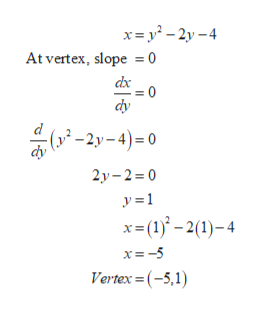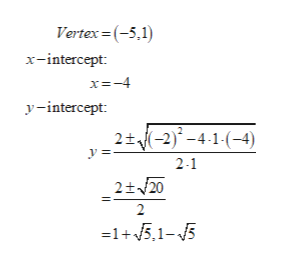# Find the equation of the axis of symmetry and the coordinates of the vertex of the parabola given by the equation.x = y2 − 2y − 4axis of symmetry       vertex    (x, y) =     Sketch the graph.

Question
2 views

Find the equation of the axis of symmetry and the coordinates of the vertex of the parabola given by the equation.

x = y2 − 2y − 4
axis of symmetry

vertex
(x, y) =

Sketch the graph.

check_circle

Step 1

Consider the following given equation,help_outlineImage Transcriptionclosex= y² - 2y – 4 At vertex, slope = 0 dx :0 dy ( -2y-4)=0 2y- 2 = 0 y =1 x=(1)° – 2(1)– 4 x= -5 Vertex =(-5,1) fullscreen
Step 2

Axis of symmetry will be along the vertex point and will be parallel to x-axis as the equation is a quadratic in terms of ‘y’. Therefore, axis of symmetry will be given by, y = 1

Step 3

To sketch the graph, calculate the intercepts of...help_outlineImage TranscriptioncloseVertex = (-5,1) x-intercept: x=-4 y-intercept: 2+(-2)* – 4-1-(-4) 2-1 2+/20 2 =1+ 5,1-5 fullscreen

### Want to see the full answer?

See Solution

#### Want to see this answer and more?

Solutions are written by subject experts who are available 24/7. Questions are typically answered within 1 hour.*

See Solution
*Response times may vary by subject and question.
Tagged in

### Equations and In-equations